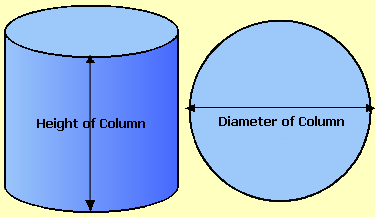Spike's Calculators

# Concrete Calculator For ColumnsCalculate the amount of concrete needed for pouring columns with results for concrete needed in cubic feet, yards, and metres. To calculate the volume of a column (cylinder), you need to know the column's diameter and height.

##### Note:
`The lineal foot amount of tubing (forming material) is rounded upwards to the nearest whole foot, but more than likely, you will end up wasting some of the material!`

### Columns Concrete Volume

Number of Columns #
Diameter in
Column Height ft in
Lineal Length of Tube lin/ft
Cubic Feet ft³
Cubic Yards yd³
Cubic Metres

#### Calculation

1. enter the number of columns
2. the diameter of the columns
3. the height of the columns

#### Results

1. the length of tubing material needed in lineal feet (rounded up to the nearest whole foot)
2. the amount of concrete needed in cubic feet
3. in cubic yards
4. in cubic metres

##### Formula's used:
```V = Π * D² ÷ 4 * h
where V = concrete volume in cubic feet
pi = 3.14159......
D = diameter
h =  height```
##### Example calculation:
```column height= 4 feet - 6 inches
diameter = 10 inches
5 columns
```

Convert 4 feet- 6 inches into inches;
`4 * 12 + 6 = 54`
`4 feet - 6 inches = 54 inches.`

Using the formula from above
`3.14159  * 10² ÷ 4  * 54 * 5 = 21205.750`
`The volume is 21205.750 cubic inches`

Convert this to cubic feet by dividing this amount by 1728 (there are 1728 cubic inches in one cubic foot);
`21205.750 / 1728 = 12.27 rounded to two decimals`
`You need 12.27 cubic feet of concrete for these five columns.`

To convert cubic feet to cubic yards multiply cubic feet * 0.037037037037037
To convert cubic feet to cubic metres multiply cubic feet * 0.028316846592
To convert cubic yards to cubic metres multiply cubic yards * 0.764554857984

### When Ordering Concrete

This concrete calculator will help you in estimating the amount of concrete needed for your project. The amount given as needed, does not include any waste. It is recommended, depending on the job you are doing, to add anywhere from an extra 4% to 10% to your concrete order to make sure you have enough concrete to finish the job. Ask when ordering the concrete. They will be able to give you a good idea of what is needed.